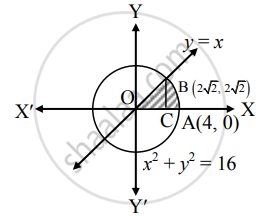# Find the area of the sector bounded by the circle x2+ y2 = 16, and the line y = x in the first quadrant - Mathematics and Statistics

Sum

Find the area of the sector bounded by the circle x2+ y2 = 16, and the line y = x in the first quadrant

#### Solution

Given equation of the circle is x2+ y2 = 16   ......(i)

and equation of the line is y = x   ......(ii)

From (i), w get

y2 = 16 − x2

∴ y = sqrt(16 - x^2)    ......(iii) .....[∵ In first quadrant, y > 0]

Substituting (ii) in (i), we get

x2 + y2 = 16

∴ 2x2 = 16

∴ x2 = 16

∴ x2 = 8

∴ x = 2sqrt(2)    .....[∵ In first quadrant, x > 0]

When x = 2sqrt(2), y= 2sqrt(2)

∴ The point of intersection is "B"(2sqrt(2), 2sqrt(2)).Required area = area of the region OCABO

= area of the region OCBO + area of the region ABCA

= int_0^(2sqrt(2)) x  "d"x + int_(2sqrt(2))^4 sqrt(16 - x^2)  "d"x    .....[From (iii) and (ii)]

= 1/2[(2sqrt(2))^2 - 0] + [4/2 sqrt(16 - 16) + 16/2 sin^-1 (1) - {(2sqrt(2))/2 sqrt(16 - 8) + 16/2 sin^-1 (1/sqrt(2))}]

= 8/2 + 16/2(pi/2) - (2sqrt(2))/2 (2sqrt(2)) - 16/2(pi/4)

= 4 + 4π − 4 − 2π

= 2π sq.units

Concept: Area Bounded by the Curve, Axis and Line
Is there an error in this question or solution?